Get inspired by the success stories of our students in IIT JAM MS, ISI  MStat, CMI MSc DS.  Learn More

# ISI MStat PSB 2009 Problem 2 | Linear Difference EquationThis is a very beautiful sample problem from ISI MStat PSB 2009 Problem 2 based on Convergence of a sequence. Let's give it a try !!

## Problem- ISI MStat PSB 2009 Problem 2

Let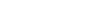be a sequence of real numbers such that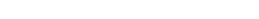for some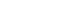(a) Show that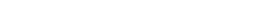(b) Hence, or otherwise, show that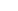converges and find the limit.

### Prerequisites

Limit

Sequence

Linear Difference Equation

## Solution :

(a) We are given thatfor someSo,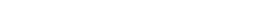---- (1)

Again using (1) we have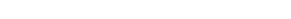.

Now putting this in (1) we have ,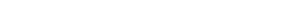.

So, proceeding like this we have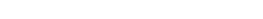for all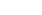and for some---- (2)

So, from (2) we have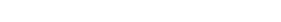,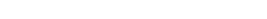and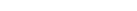Adding all the above n equation we have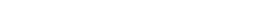Hence ,(proved ) .

(b) As we now have an explicit form of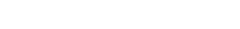----(3)

Hence from (3) we can sayis bounded and monotonic ( verify ) so , it's convergent .

Now let's take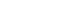both side of (3) we get ,.

Since ,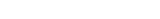as ,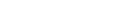.

## Food For Thought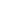and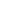are two natural number and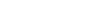and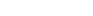are two sets of positive real numbers such that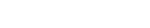=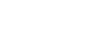for all natural number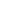Then prove that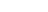and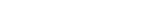.

## Subscribe to Cheenta at Youtube

This is a very beautiful sample problem from ISI MStat PSB 2009 Problem 2 based on Convergence of a sequence. Let's give it a try !!

## Problem- ISI MStat PSB 2009 Problem 2

Letbe a sequence of real numbers such thatfor some(a) Show that(b) Hence, or otherwise, show thatconverges and find the limit.

### Prerequisites

Limit

Sequence

Linear Difference Equation

## Solution :

(a) We are given thatfor someSo,---- (1)

Again using (1) we have.

Now putting this in (1) we have ,.

So, proceeding like this we havefor alland for some---- (2)

So, from (2) we have,andAdding all the above n equation we haveHence ,(proved ) .

(b) As we now have an explicit form of----(3)

Hence from (3) we can sayis bounded and monotonic ( verify ) so , it's convergent .

Now let's takeboth side of (3) we get ,.

Since ,as ,.

## Food For Thoughtandare two natural number andandare two sets of positive real numbers such that=for all natural numberThen prove thatand.

## Subscribe to Cheenta at Youtube

This site uses Akismet to reduce spam. Learn how your comment data is processed.

### Knowledge Partner